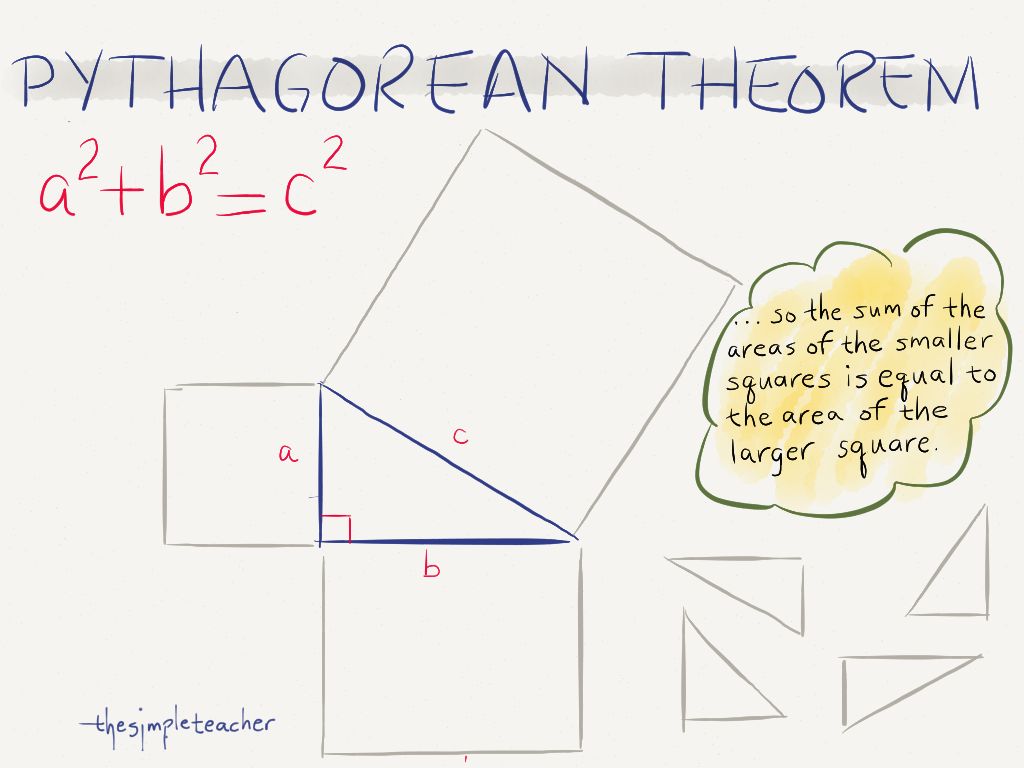cyberoffice‎ > ‎

### pythagorean theorem

 Basic Understanding: The Pythagorean Theorem, a2 + b2 = c2, shows the relationship between the sides of a right triangle. In the equation, a and b represent the length of the legs of the right triangle and c represents the length of the hypotenuse of the right triangle.Pythagorean Theorem Water Demo Construct a Right Triangle: Use the right angle construction to create a right triangle, then label the legs and hypotenuse with a, b, and c respectively. References "Pythagorean Theorem Water Demo." From YouTube https://www.youtube.com/watch?v=CAkMUdeB06o. Page, John D. "Constructing a 90o Angle." From Math Open Reference Sample LON-CAPA Problems Finding the Length of the Hypotenuse. Draw a right triangle, then label one leg with 16 cm and the other leg with 40 cm. Find the length of the hypotenuse to the nearest tenth. The length of the hypotenuse is __________ cm. Hint (appears after student has attempted the problem): Draw and label a right triangle. Substitute the values given into the Pythagorean Theorem. Solve for the length of the hypotenuse. Finding the Length of a Leg. Draw a right triangle, then label one leg with 7 cm and the hypotenuse with 38 cm. Find the length of the other leg to the nearest tenth. The length of the hypotenuse is __________ cm. Hint: Draw and label a right triangle. Substitute the values given into the Pythagorean Theorem. Solve for the length of the leg. Escalator Geometry. The American Society of Mechanical Engineers Safety Code for Elevators and Escalators requires the angle of inclination for escalators to be a maximum of 30o. The distance from work point to work point of an escalator is typically equal to the distance between floors x 1.73205. If the distance between floors in a new shopping mall is 24 feet, then what is the distance escalator travels to the nearest foot? The length of the escalator ride is __________ feet. Hint: Imagine a 30o-60o-90o triangle laying down on its longest leg with its shortest leg pointing up toward the second floor of the mall. Transformations. Draw a rectangle with vertices at A(0,0), B(0,13), C(9,13), and D(9,0) on a coordinate graph, then rotate the rectangle using the rule, R(x,y) → (-y,x). Find the length of the line segment connecting C to C'. Round your answer to the nearest tenth. The length of the line segment is __________ units. Hint: Draw and label a the rectangle. Rotate the rectangle using the rule: R(x,y) → (-y,x). Draw a line segment from C to C'. Sketch a right triangle using the line segment as the hypotenuse. Use the Pythagorean Theorem to find the length of the line segment connecting points C to C'. Answer Key